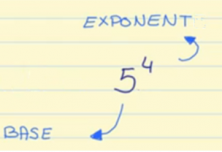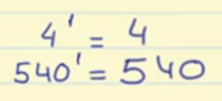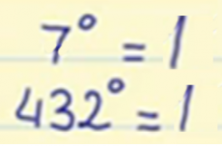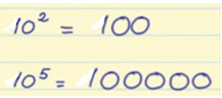Smartick is a fun way to learn math!Jun29

In this post, we are going to learn what exponents are and how to calculate them.

Exponents are used to simplify multiplication problems where the same number is multiplied.

For example:

## 5 x 5 x 5 x 5

We are multiplying the number 5 four times. In order to write it in powers, we first write down the number 5 and above on the left side, we write a small number 4.The number 5 is the base, which is the number that is multiplied. The number 4 is the exponent, which is the number of times the base is multiplied.

It’s read like this: 5 to the 4th power.

#### Special Cases of Exponents:

• Exponent of 1: Any number to the power of 1 will always be the same number.• Exponent of 0: Any number to the power of zero will always be 1.• Base 10: The exponent indicates the number of zeros that the answer will have.If you want to see a more detailed explanation with more examples of powers, you can check out a similar post: Powers in Math.

And of course if you want to learn more math, register at Smartick for a 15-day free trial!